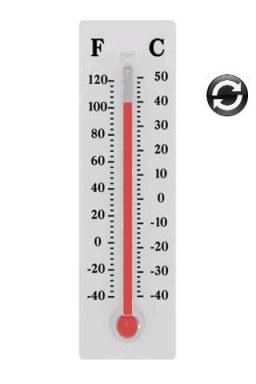# Temperature linear fit

At 2:00 a.m. the temperature was -7°F. at noon the temperature was 18°F. What expression represents the increase in temperature?

Result

e = (Correct answer is: 2.5*T-12)### Step-by-step explanation:Did you find an error or inaccuracy? Feel free to write us. Thank you!

Showing 1 comment:
Meldrin
On December 25,2009 the temperature at noon was approximated by T + 2 H = - 2° C.Estimate the temperature at 2 pm today?

Tips for related online calculators
Do you have a linear equation or system of equations and looking for its solution? Or do you have a quadratic equation?
Do you want to convert time units like minutes to seconds?